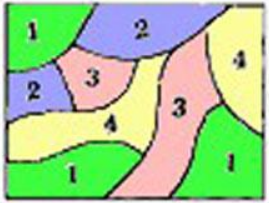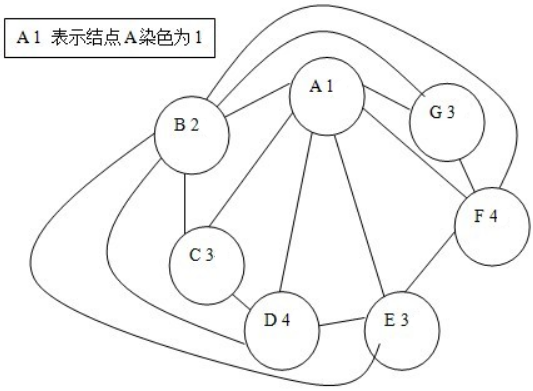# POJ 1129 - Channel Allocation

## 解题思路

``since the repeaters lie in a plane, the graph formed byconnecting adjacent repeaters does not have any line segments that cross.``

## 提示

“至少一条边重合”同时也隐含了“任意边（线段）不正规相交。``````N=7

A:BCDEFG

B:ACDEFG

C:ABD

D:ABCE

E:ABDF

F:ABEG

G:ABF``````## AC 源码

### 解题方法一：四色定理剪枝的搜索

``````/*四色定理*/

//Memory Time
//184K   0MS

#include<iostream>
using namespace std;

typedef class
{
public:
int next;  //直接后继
int pn;   //next[]指针（后继个数）
}point;

int main(int i,int j,int k)
{
int n;
while(cin>>n)
{
if(!n)
break;

getchar();  //n的换行符

point* node=new point[n+1];  //结点

/*Structure the Map*/

for(i=1;i<=n;i++)
{
getchar();  //结点序号
getchar();  //冒号

if(node[i].pn<0)   //初始化指针
node[i].pn=0;

char ch;
while((ch=getchar())!='\n')
{
j=ch%('A'-1);   //把结点字母转换为相应的数字，如A->1  C->3
node[i].next[ ++node[i].pn ]=j;
}
}

int color={0};  //color[i]为第i个结点当前染的颜色，0为无色（无染色）
color=1;  //结点A初始化染第1种色
int maxcolor=1;  //当前已使用不同颜色的种数

for(i=1;i<=n;i++)  //枚举每个顶点
{
color[i]=n+1;  //先假设结点i染最大的颜色
bool vist={false};  //标记第i种颜色是否在当前结点的相邻结点染过
for(j=1;j<=node[i].pn;j++) //枚举顶点i的所有后继
{
int k=node[i].next[j];
if(color[k])  //顶点i的第j个直接后继已染色
vist[ color[k] ]=true;  //标记该种颜色
}
for(j=1;i<=n;j++)  //从最小的颜色开始，枚举每种颜色
if(!vist[j] && color[i]>j) //注意染色的过程是一个不断调整的过程，可能会暂时出现大于4的颜色
{                          //因此不能单纯枚举4种色，不然会WA
color[i]=j;
break;
}

if(maxcolor<color[i])
{
maxcolor=color[i];
if(maxcolor==4)   //由四色定理知，最终完成染色后，图上最多只有四种颜色
break;        //因此当染色过程出现结点的颜色为4时，就可以断定最少要用4种颜色染色
}
}

if(maxcolor==1)
cout<<1<<" channel needed."<<endl;
else
cout<<maxcolor<<" channels needed."<<endl;

delete node;
}
return 0;
}``````

### 解题方法二：暴力搜索

``````/*暴力搜索*/

//Memory Time
//184K   0MS

#include<iostream>
using namespace std;

typedef class
{
public:
int next;  //直接后继
int pn;   //next[]指针（后继个数）
}point;

int main(int i,int j,int k)
{
int n;
while(cin>>n)
{
if(!n)
break;

getchar();  //n的换行符

point* node=new point[n+1];  //结点

/*Structure the Map*/

for(i=1;i<=n;i++)
{
getchar();  //结点序号
getchar();  //冒号

if(node[i].pn<0)   //初始化指针
node[i].pn=0;

char ch;
while((ch=getchar())!='\n')
{
j=ch%('A'-1);   //把结点字母转换为相应的数字，如A->1  C->3
node[i].next[ ++node[i].pn ]=j;
}
}

int color={0};  //color[i]为第i个结点当前染的颜色，0为无色（无染色）
color=1;  //结点A初始化染第1种色
int maxcolor=1;  //当前已使用不同颜色的种数

for(i=1;i<=n;i++)  //枚举每个顶点
{
color[i]=n+1;  //先假设结点i染最大的颜色
bool vist={false};  //标记第i种颜色是否在当前结点的相邻结点染过
for(j=1;j<=node[i].pn;j++) //枚举顶点i的所有后继
{
int k=node[i].next[j];
if(color[k])  //顶点i的第j个直接后继已染色
vist[ color[k] ]=true;  //标记该种颜色
}
for(j=1;i<=n;j++)  //从最小的颜色开始，枚举每种颜色，最终确定结点i的染色
if(!vist[j] && color[i]>j)
{
color[i]=j;
break;
}

if(maxcolor<color[i])
maxcolor=color[i];
}

if(maxcolor==1)
cout<<1<<" channel needed."<<endl;
else
cout<<maxcolor<<" channels needed."<<endl;

delete node;
}
return 0;
}``````

目录